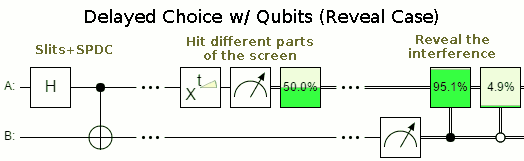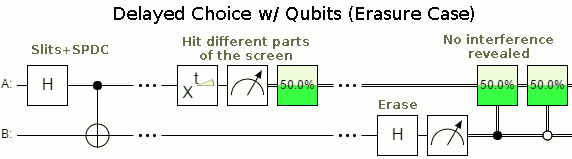# What is the quantum circuit equivalent of a (delayed choice) quantum eraser?

Quantum computers are efficiently able to simulate any other quantum system. Hence there must be some sort of equivalent of a (possibly simulated) quantum eraser setup. I would like to see such an equivalent drawn as a quantum circuit, ideally in the variant of a delayed choice quantum eraser.

One (quantum) experimental realization of a quantum eraser is this: You create a double slit interference experiment where you obtain which-way information by "doubling" photons in front of each slit using spontaneous parametric down conversion (the physics of which are not important for my argument, the point being that we have a new photon we can measure to obtain which-way information). The interference pattern naturally disappears, unless we build a quantum eraser: If the two "doubled" photons carrying the which-way information are superimposed via a 50-50 beamsplitter in such a manner that the which-way information can no longer be measured, the interference pattern reappears. Curiously, this is experimentally the case even if this superimposing happens after the interference pattern is recorded.

I seem to be unable to find a convincing equivalence for the interference pattern and for the quantum eraser in simple qubit gates. But I would love to make the thought (and ideally, the real) experiment on a quantum computer, too. What program (quantum circuit) would I need to run on a quantum computer to do that?

I will try to translate the Kim et. al. experiment from an optics description into a quantum information description. Here is the experimental setup as you find it in the linked wikipedia article:We associate the blue path with $|0\rangle$ and the red with $|1\rangle$. The double slit can be described by a Hadamard gate. The BBO corresponds to a CNOT-gate. The state after the BBO is $\frac{1}{\sqrt{2}}(|00\rangle + |11\rangle)$. There is a phase $\varphi$ depending on the position $x$ of detector $D_0$, which corresponds to a phase gate $R_\varphi=\operatorname{diag}(1,e^{i\varphi})$. Finally superposing the beams on $D_0$ corresponds to another Hadamard gate and the measurement of $D_0$ can be seen to project onto $|0\rangle$. The complete circuit looks like this:The state before the measurement is: $$\frac{1}{2}(|00\rangle+|10\rangle + e^{i\varphi}|01\rangle - e^{i\varphi}|11\rangle)=\frac{1}{2}(((1+e^{i\varphi})|0\rangle+(1-e^{i\varphi})|1\rangle)|+\rangle + ((1-e^{i\varphi})|0\rangle +(1+e^{i\varphi})|1\rangle)|-\rangle)$$
Let's look at the probability to measure the first photon in $D_0$ ($|0\rangle\langle 0|$).
If we measure the second in z-basis ($D_3$ and $D_4$), the probability for a click in $D_0$ is $\frac{1}{2}$ (the post-measurement state is $|\pm\rangle$). This is independent of the phase: no interference here. For the x-basis ($D_1$ and $D_2$) the probability for a click at $D_0$ is $\frac{1}{2}(1\mp \cos \varphi)$, so here we see the interference. Whether we see interference or not depends on the basis choice on the second system, which can be delayed. Of course we need to know the outcome, so faster than light communication is not possible with this setup.

• Awesome, thank you! Don't worry about the circuit; the description is so clear that the circuit can easily be drawn following it.
– user1039
Apr 2, 2018 at 19:56
• Even so ^, I think having the circuit would be a nice addition.. :-)
– Kiro
Apr 2, 2018 at 20:57
• @Kiro: I agree and include the diagram in the answer. Apr 2, 2018 at 21:48

Quirk includes a mockup of the delayed choice eraser as an example circuit:Any situation where you create an entangled state, and then switch between measuring in the same basis on both sides or a different basis will show the effect. You can reduce it down to fewer qubits if you're willing to give up the more visually direct waves in the output expectation:In fact you can even make classical analogues:

Bob has a six-sided die, a two-sided coin, and a small empty box to put the coin in. He rolls the die, gets a result between 1 and 6, and places the coin in the box in a way that depends on the die roll. If the die roll was even, the coin is placed heads up. If the die roll was odd, the coin is placed tails up. Bob then writes down his die roll, and carefully hands the box to Alice.

Alice now has to decide to either a) just open the box or b) shake the box before opening it. (Shaking the box randomizes the coin.) Once the box is open, she writes down whether the coin was face up or face down, and also writes down whether or not she shook the box.• Would it be possiblle for the delayed choice experiment be reformulated as a grover search circuit? Or what the challenges in doing/not being able to do it in a grover way be? Dec 29, 2020 at 21:53